# 1 信息存储

## 1.2 字数据大小

short与long两个限定符的引入可以为我们提供满足实际需要的不同长度的整形数。int通常代表特定机器中证书的自然长度。short类型通常为16位，long类型通常为32位，int类型可以为16位或32位。各编译器可以根据硬件特性自主选择合适的类型长度，但要遵循下列限制：short与int类型至少为16位，long类型至少为32位，并且short类型不得长于int类型，而int类型不得长于long类型。————《C程序设计语言》

[signed] charunsigned char11
shortunsigned short22
intunsigned int44
longunsigned long48
long longunsigned long long88
int8_tuint8_t11
int16_tuint16_t22
int32_tuint32_t44
int64_tuint64_t88
char *48
float44
double88

# 2 整数表示与计算

## 2.3 整型计算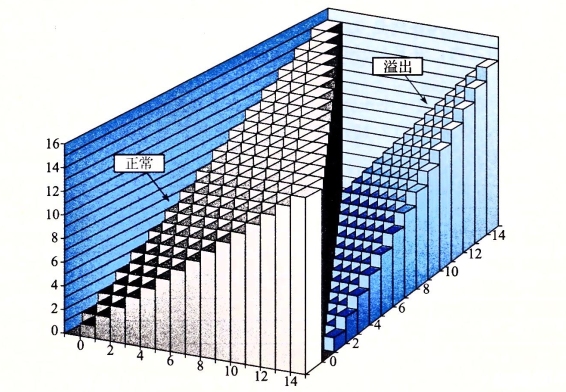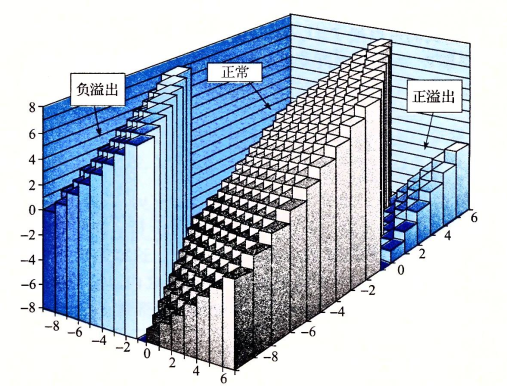# 3 浮点数表示与计算

## 3.1 浮点型的编码规则

IEEE制定了浮点型的编码规则，使得浮点数能够更加精确的表示实数，该标准使用 V = ( − 1 ) s × M × 2 E V=(-1)^s\times M\times 2^E 的形式来表示一个数，你可以理解为二进制的科学计数法的一种变体：

• 符号(sign)： s s 决定了这个数是正数( s = 0 s=0 )还是负数( s = 1 s=1 )

• 阶码(exponet)：E表示阶数，这里E是一个有符号整型，但是并不采用补码编码，而是另外一种方式，这里 E = e x p − B a i s E=exp-Bais 或者 E = 1 − B i a s E=1-Bias ，具体哪一种由下一节介绍。其中 e x p = e k − 1 e k − 2 . . . e 1 e 0 exp=e_{k-1}e_{k-2}...e_1e_0 代表无符号数， B i a s Bias 表示偏置，是一个值为 2 k − 1 − 1 2^{k-1}-1 的数(在float中k=9，Bais等于127，double中k=11，Bais为1023)。

• 尾数(significand)：M是一个二进制小数，它的范围是1~2- ϵ \epsilon 或者0~1- ϵ \epsilon ，具体是哪一种范围由下一节介绍。M由原实数化为科学计数法的二进制的小数部分编码(frac字段)

下面用CSAPP中的图直观地表示浮点型的构成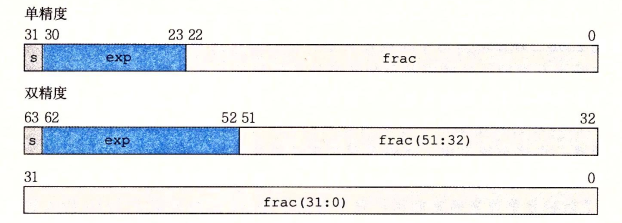## 3.2 浮点数的分类表示

• 规格化的值：表示绝对值比较大的浮点数(绝大部分数，我们可以认为正常的数都在这个范围)，在这一部分，我们的 E = e x p − B i a s E=exp-Bias ，而尾数M的范围1~2- ϵ \epsilon ，而frac字段则实际上是M的小数部分，即 M = 1 + f r a c M=1+frac ，故 0 ≤ f r a c < 1 0\le frac<1
• 非规格化的值：表示趋于零的实数，在这一部分，我们的 E = 1 − B i a s E=1-Bias ，而尾数的范围是0~1- ϵ \epsilon ，此时frac字段依然表示M的小数部分，但是由于M的整数部分为0，故 M = f r a c M=frac
• 特殊值：这一类是指阶码全部为1的时候，可以分为两类，无穷大和NAN(Not A Number)。当阶码全部为1，小数部分(frac字段)全部为0时，表示正无穷，我们可以感性地理解为浮点数表示不了这么大的数字，所以它是正无穷。当阶码全部为1，小数部分(frac字段)不为0时，则表示NAN，我们可以感性地认为由于这个数的小数部分没有确定，所以可以有很多取值的可能性，因此是Not A Number。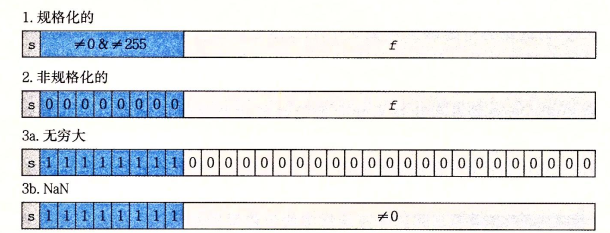V = 10.62 5 ( 10 ) V=10.625_{(10)} ，我们先将其化为而二进制，则 V = 1010.10 1 ( 2 ) V=1010.101_{(2)} ，写成IEEE标准则是 V = ( − 1 ) 0 × 1.010101 × 2 3 V=(-1)^0\times 1.010101\times 2^3 。显然 s = 0 , e x p = 3 ( 10 ) + B i a s ( f l o a t ) = 1000001 0 ( 2 ) , f r a c = 010101 s=0,exp=3_{(10)}+Bias_{(float)}=10000010_{(2)},frac=010101 ，这里要注意，frac去掉了整数部分的1为了最大限度的表示小数。

U = 0.937 5 ( 10 ) U=0.9375_{(10)} ，我们先将其转化为二进制，则 U = 0.111 1 ( 2 ) U=0.1111_{(2)} ，写成IEEE标准则是 U = ( − 1 ) 0 × 1.111 × 2 − 1 U=(-1)^0\times 1.111\times 2^{-1} 。显然 s = 0 , e x p = − 1 ( 10 ) + B i a s ( f l o a t ) = 12 6 ( 10 ) = 111111 0 ( 2 ) , f r a c = 111 s=0,exp=-1_{(10)}+Bias_{(float)}=126_{(10)}=111 1110_{(2)},frac=111

000…000…00

101…110…00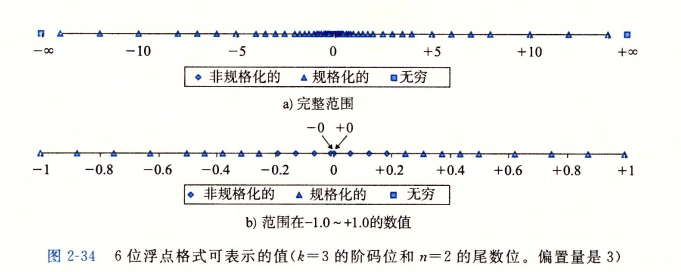## 3.4 浮点数的计算

02-1096
10-1934207-1794
10-2531
12-1744
10-196116
03-089057
10-095926
07-093万+
12-08
03-09
06-03
12-052万+
12-262万+
03-25
12-03
©️2020 CSDN 皮肤主题: 游动-白 设计师:白松林